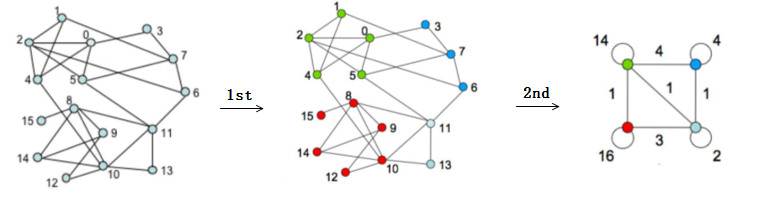# GraphX 在图数据库 Nebula Graph 的图计算实践安祺2020-11-10特性讲解## 二、算法应用

### PageRank

PageRank 是谷歌提出的用于解决链接分析中网页排名问题的算法，目的是为了对互联网中数以亿计的网页进行排名。

#### PageRank 简介

1. 数量假设：如果一个网页 A 被很多其他网页链接到，则该网页比较重要；
2. 质量假设：如果一个很重要的网页链接到网页 A，则该网页的重要性会被提高。

#### 算法思路

GraphX 的 PageRank 算法是基于 Pregel 计算模型的，该算法流程包括 3 步骤：

1. 为图中每个节点（网页）设置一个同样的初始 PageRank 值；
2. 第一次迭代：沿边发送消息，每个节点收到所有关联边上对点的信息，得到一个新的 PageRank 值；
3. 第二次迭代：用这组新的 PageRank 按不同算法模式对应的公式形成节点自己新的 PageRank。

### Louvain 社区发现

Louvain 是用来进行社会网络挖掘的社区发现算法，属于图的聚类算法。

#### Louvain 算法介绍

Louvain 是基于模块度（Modularity）的社区发现算法，通过模块度来衡量一个社区的紧密程度。如果一个节点加入到某一社区中会使得该社区的模块度相比其他社区有最大程度的增加，则该节点就应当属于该社区。如果加入其它社区后没有使其模块度增加，则留在自己当前社区中。

##### 模块度

$$Q = \frac{1}{2m} \times \sum_{i,j}[A_{ij} - \frac{k_ik_j}{2m}]\delta(c_i,c_j) \ 条件：\delta(u,v) = \begin{cases} 1 &\text{when u == v} \ 0 &\text {}else \end{cases}$$

$A_{ij}$：节点 i 和节点 j 之间边的权重 $K_i$ ：所有与节点 i 相连的边的权重之和 $C_i$ ：节点 i 所属的社区 $m$ ： 图中所有边的权重之和

$Q = \frac{1}{2m} \times \sum{i,j}[A{i,j} - \frac{k_ik_j}{2m}]\delta(c_i,c_j) \ = \frac{1}{2m}[\sum{i,j}A{i,j} - \frac{\sum_ik_i \sum_jk_j}{2m}]\delta(c_i,c_j) \ = \frac{1}{2m} \sum_c[\sum{in} - \frac{{\sum{tot}}^{2}}{2m}]$

$\Sigma_{in}$ 表示： 社区 c 内的边的权重之和 $\Sigma_{k_n}$ 表示： 所有与社区 c 内节点相连的边的权重之和（因为 i 属于社区 c）包括社区内节点与节点 i 的边和社区外节点与节点 i 的边。 $\Sigma_{k_j}$ 表示： 所有与社区 c 内节点相连的边的权重之和（因为 j 属于社区 c）包括社区内节点与节点 j 的边和社区外节点与节点 j 的边。 _$\Sigma{tot}$ _代替_ $\Sigma{k_i}$_ 和 $\Sigma_{k_j}$。（即社区 c 内边权重和 + 社区 c 与其他社区连边的权重和）

$\Delta{Q} = [\frac{\sum{in} + k{i,in}}{2m} -({\frac{\sum{tot} + k_i}{2m}})^{2}] - [\frac{\sum{in}}{2m} - (\frac{\sum_{tot}}{2m})^{2} - (\frac{k_i}{2m})^{2}] \ = [\frac{k{i,in}}{2m} - \frac{\sum{tot}k_i}{2m^2}]$

$k_{i,in}$： 社区内所有节点与节点 i 连边权重之和（对应新社区的实际内部权重和乘以 2，因为 $k_{i,in}$ 对于社区内所有的顶点 i，i 关联的每条边其实被计算了两次） $K_i$： 所有与节点 i 相连的边的权重之和 故实现算法时只需求 $[k{i,in} - \sum{tot} \times \frac{k_i}{m}]$ 即可。

• 金融风控

• 社交网络

• 推荐系统

#### Louvain 算法思路

Louvain 算法包括两个阶段，其流程就是这两个阶段的迭代过程。## 三、算法实践

### 演示环境

• 三台虚拟机，环境如下：
• Cpu name：Intel(R) Xeon(R) Platinum 8260M CPU @ 2.30GHz
• Processors：32
• CPU Cores：16
• Memory Size：128G
• 软件环境
• yarn V2.10.0：三个节点集群
• Nebula Graph V1.1.0：分布式部署，默认配置

### 测试数据

1. 创建图空间
CREATE SPACE algoTest(partition_num=100, replica_factor=1);

1. 创建点边 Schema
CREATE TAG PERSON()
CREATE EDGE FRIEND(likeness double);

1. 导入数据

1. 测试结果

Spark 任务的资源分配为 --driver-memory=20G --executor-memory=100G --executor-cores=3

• PageRank 在一亿数据集上的执行时间为 21min（PageRank 算法执行时间）
• Louvain 在一亿数据集上的执行时间为 1.3h（Reader + Louvain 算法执行时间）

### 如何使用 Nebula Graph 的算法

1. 下载 nebula-algorithm 项目并打成 jar 包
$git clone git@github.com:vesoft-inc/nebula-java.git$ cd nebula-java/tools/nebula-algorithm
\$ mvn package -DskipTests

1. 配置项目中的 src/main/resources/application.conf
{
# Spark relation config
spark: {
app: {
# not required, default name is the algorithm that you are going to execute.
name: PageRank

# not required
partitionNum: 12
}

master: local

# not required
conf: {
driver-memory: 8g
executor-memory: 8g
executor-cores: 1g
cores-max:6
}
}

# Nebula Graph relation config
nebula: {
user: root
pswd: nebula
space: algoTest
# partition specified while creating nebula space, if you didn't specified the partition, then it's 100.
partitionNumber: 100
# nebula edge type
labels: ["FRIEND"]

hasWeight: true
# if hasWeight is true，then weightCols is required， and weghtCols' order must be corresponding with labels.
# Noted: the graph algorithm only supports isomorphic graphs,
#        so the data type of each col in weightCols must be consistent and all numeric types.
weightCols: [“likeness”]
}

algorithm: {
# the algorithm that you are going to execute，pick one from [pagerank, louvain]
executeAlgo: louvain
# algorithm result path
path: /tmp

# pagerank parameter
pagerank: {
maxIter: 20
resetProb: 0.15  # default 0.15

}

# louvain parameter
louvain: {
maxIter: 20
internalIter: 10
tol: 0.5
}
}
}

1. 确保用户环境已安装 Spark 并启动 Spark 服务

2. 提交 nebula-algorithm 应用程序：

spark-submit --master xxx --class com.vesoft.nebula.tools.algorithm.Main /your-jar-path/nebula-algorithm-1.0.1.jar -p /your-application.conf-path/application.conf


## 推荐阅读